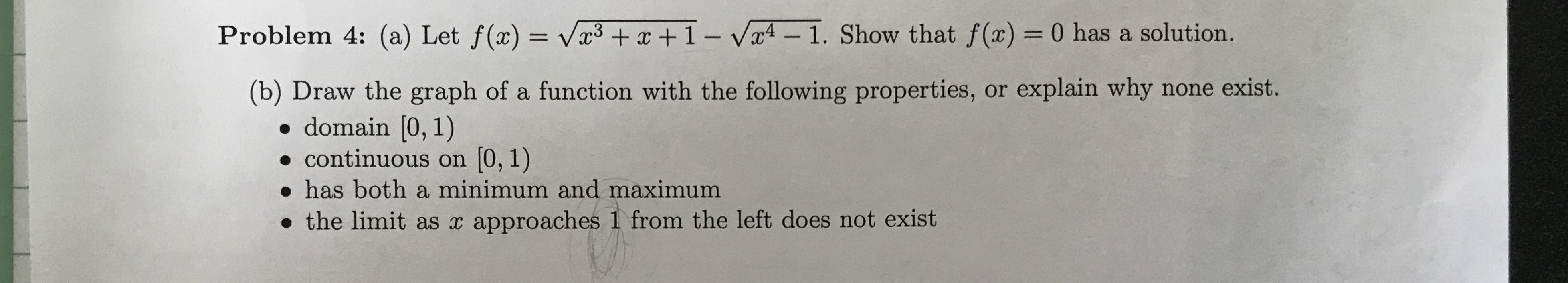# Problem 4: (a) Let f(x) = V+ x + 1 - Va4- 1. Show that f(x) = 0 has a solution. (b) Draw the graph of a function with the following properties, or explain why none exist. domain [0, 1) continuous on 0,1) has both a minimum and maximum the limit as x approaches 1 from the left does not exist

Questionhelp_outlineImage TranscriptioncloseProblem 4: (a) Let f(x) = V+ x + 1 - Va4- 1. Show that f(x) = 0 has a solution. (b) Draw the graph of a function with the following properties, or explain why none exist. domain [0, 1) continuous on 0,1) has both a minimum and maximum the limit as x approaches 1 from the left does not exist fullscreen